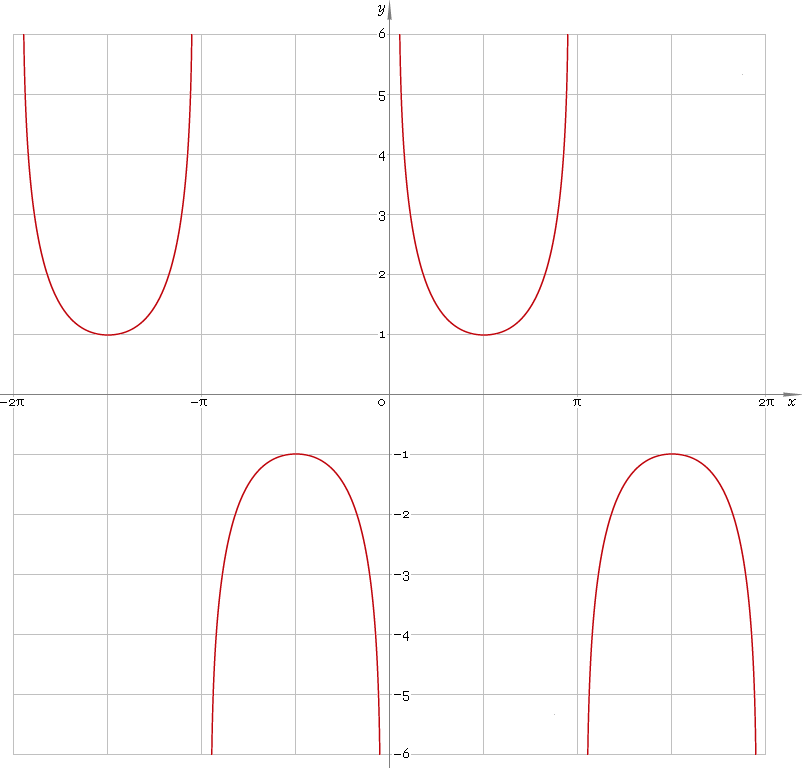The Art of Interface

# csc or cosec — trigonometric cosecant function

Category. Mathematics.

Abstract. Trigonometric cosecant: definition, graph, properties, identities and table of values for some angles.

## 1. Definition

Cosecant of the angle is ratio of the hypotenuse to the opposite leg.

## 2. Graph

Cosecant is 2π periodic function defined everywhere on real axis, except its singular points πn, n=0, ±1, ±2, ... — so, function domain is (πn, π(n + 1)), n∈N. Its stalactite-stalagmite graph is depicted below — fig. 1.Fig. 1. Graph of the cosecant function y = cscx.

Function codomain is entire real axis with gap in the middle: (−∞, −1]∪[1, +∞).

## 3. Identities

Base:

csc−2φ + sec−2φ = 1

and its consequences:

cscφ = ±1 /√(1 − cos2φ)
cscφ = ±√(1 + tan2φ) / tanφ
cscφ = ±√(1 + cot2φ)
cscφ = ±secφ /√(sec2φ − 1)

By definition:

cscφ ≡ 1 /sinφ

Properties — symmetry, periodicity, etc.:

csc−φ = −cscφ
cscφ = csc(φ + 2πn), where n = 0, ±1, ±2, ...
cscφ = csc(π − φ)
cscφ = −csc(π + φ)
cscφ = sec(π/2 − φ)

Sum of angles:

csc(φ + ψ + χ) = secφ secψ secχ / (tanφ + tanψ + tanχ − tanφ tanψ tanχ)

Some angles:

Angle φValue cscφ
π/12√6 + √2
π/10√5 + 1
π/8√(4 + 2√2)
π/62
π/5√(50 + 10√5) /5
π/4√2
3π/10√5 − 1
π/32√3 /3
3π/8√(2 − √2)
2π/5√(50 − 10√5) /5
5π/12√6 − √2
π/21
Table 1. Cosecant for some angles.

## 4. Support

Trigonometric cosecant function csc or cosec is supported in:

Trigonometric cosecant function of the complex argument csc or cosec is supported in:

## 5. How to use

To calculate cosecant of the number:

``csc(-1);``

To calculate cosecant of the current result:

``csc(Rslt);``

To calculate cosecant of the angle φ in memory:

``csc(Mem[φ]);``